# First attempt at using GNU Radio blocks from IPython¶

This is my first attempt at using GNU Radio blocks from within IPython. I'm going to jump right in and try to do a weather radio receiver with the channel extractor and FM decoder I put together in my last bit of work. As last time, the output will be stuck into a raw audio sample file, which I will play with aplay(1) on the command-line. I could just string together all the GNU Radio components for this (as you'd do in GRC), but that's less interesting to me right now.

My main goal in this is to get familiar with how to string blocks together, but also how to get the data out of blocks and into "proper" code.

As an aside: working with GRC is like working with little minicircuits modules in a lab. It's very neat, and very tidy, but also very constraining. If what you want to do can be done with those modules, you're in a good place. On the other hand, what most software people are used to is something more akin to "Experimental Methods in RF Design." EMRFD is a great book for hobbyist/amateur radio types, and is full of small circuit designs that can be slapped together on a piece of bare copper board. It's sort of modular, but every module is something you built, and therefore you can tap signals in anywhere you'd like.

Anyway. This is my first attempt to move from my EM:RFD RTL work into something a bit more pleasantly componentized (ie: using gnuradio blocks).

The general idea is to set up a UHD USRP interface, slurp off a bunch of samples, and then do a "manual" decode of the FM weather radio signal.

In :
%pylab inline

import scipy
import scipy.signal

Populating the interactive namespace from numpy and matplotlib


In :
Fc=int(162.42e6)
Fs=int(50e3)
gain=50
N = int(1e6)

In :
# Set up our flow graph
#
tb = gr.top_block()

# Set up the USRP for input
usrp_source = uhd.usrp_source(",", uhd.stream_args(cpu_format="fc32", channels=))
usrp_source.set_samp_rate(Fs)
usrp_source.set_center_freq(Fc, 0)
usrp_source.set_gain(gain, 0)

# Let's try to flush out the first bunch of samples

# Limit ourselves to N samples

# And a sink to dump them into
sink = blocks.vector_sink_c()

tb.connect(usrp_source, skip_head, head, sink) # Can use the handy serial connect method here

In :
tb.run()
tb.stop()

In :
x = np.array(sink.data())
plot(x[0:100])

Out:
[<matplotlib.lines.Line2D at 0x7fd584d7aed0>]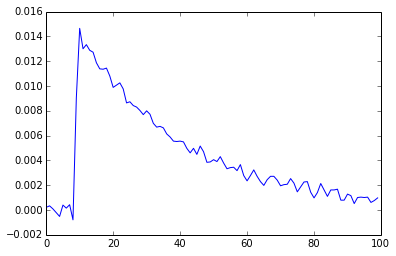In :
specgram(x, Fs=Fs); None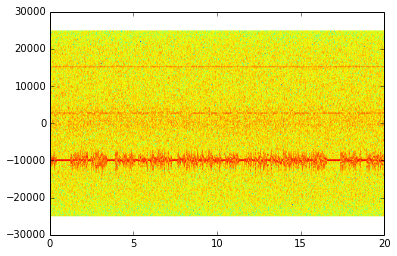In :
psd(x, Fs=Fs); None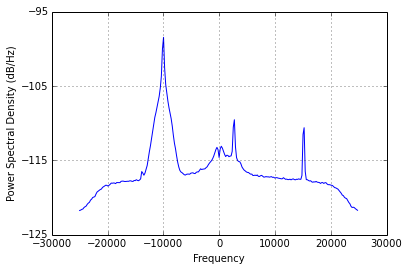In :
def extract_channel(f_sample, f_center, f_bw, x):
"""Extract a channel of width f_bw at f_center, from f_sample

Returns Fs,y, the new sample rate and an array of complex samples
"""

# Cribbing from the GNURadio xlate, we use their block diagram

my_state = {} # cheating backchannel for debug

# Create a LPF for our target bandwidth
n_taps = 100 # XXX Total random shot in the dark
r_cutoff = float(f_bw)/f_sample
base_lpf = scipy.signal.remez(n_taps, [0, r_cutoff, r_cutoff*2, 0.5], [1,0])

# Shift this LPF up to our center frequency
fwT0 = 2.0*np.pi*f_center/f_sample

lpf = base_lpf * np.exp(1.0j*fwT0 * np.arange(0, n_taps))

dec_rate = int(f_sample / (2*f_bw))

x_filtered = scipy.signal.lfilter(lpf, 1.0, x)

dec_is = np.arange(0, len(x_filtered), dec_rate)
y = x_filtered[dec_is]
y *= np.exp(-1.0j*fwT0*dec_is)

my_state["n_taps"] = n_taps
my_state["r_cutoff"] = r_cutoff
my_state["lpf"] = lpf
my_state["base_lpf"] = base_lpf
my_state["fwT0"] = fwT0
my_state["dec_rate"] = dec_rate
# my_state["dec_is"] = dec_is
# my_state["x_filtered"] = x_filtered

return f_sample/dec_rate, y, my_state

xp = x[1:] * np.conj(x[:-1])
retval = gain * np.arctan2(np.imag(xp), np.real(xp))
lpf = scipy.signal.remez(30, [0.05, 0.25, 0.27, 0.5], [1,0])
retval = scipy.signal.lfilter(lpf, 1.0, retval)
return retval


In :
Fs_new, y, _ = extract_channel(Fs, -10000, 5000, x)
specgram(y, Fs=Fs_new)
Fs_new

Out:
10000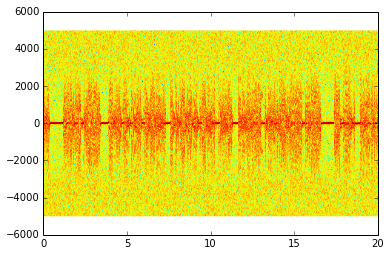In :
w = decode_quad(y, 10.0)

In :
plot(w[0:1000])

Out:
[<matplotlib.lines.Line2D at 0x7fd584c29410>]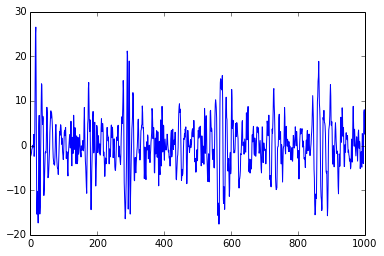In :
(w*500).astype("int16").tofile("wx.raw")
# aplay -r 10k -f S16_LE -t raw -c 1 < wx.raw

In :
specgram(w[0:10000], Fs=Fs_new); None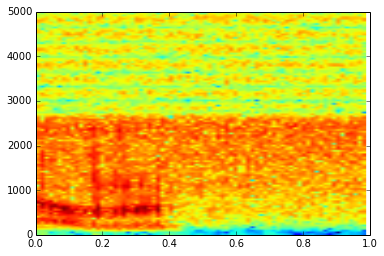In :
from subprocess import call
call("aplay -r 20k -f S16_LE -t raw -c 1 wx.raw".split(" "))

Out:
0

In :
max(abs(w)*500)

Out:
13594.236143314036

In :
audio_rate = 22050 # close enough for now...

file_source = blocks.file_source(gr.sizeof_short, "wx.raw", repeat=False)

throttle = blocks.throttle(gr.sizeof_short, audio_rate)

stf = blocks.short_to_float(scale=10000.0) # the scale here is exactly backwards of what I'd expect...

v_sink = blocks.vector_sink_f()

audio_sink = audio.sink(audio_rate)

ab = gr.top_block()
ab.connect(file_source, throttle, stf, audio_sink)
ab.connect(stf, v_sink)

ab.run()

In :
u = np.array(v_sink.data())

plot(u[0:1000])

Out:
[<matplotlib.lines.Line2D at 0x7fd586f0bfd0>]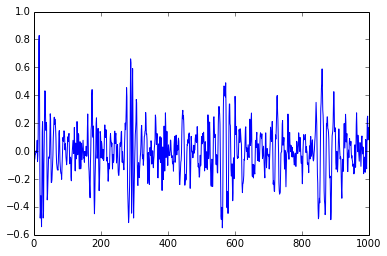In []: